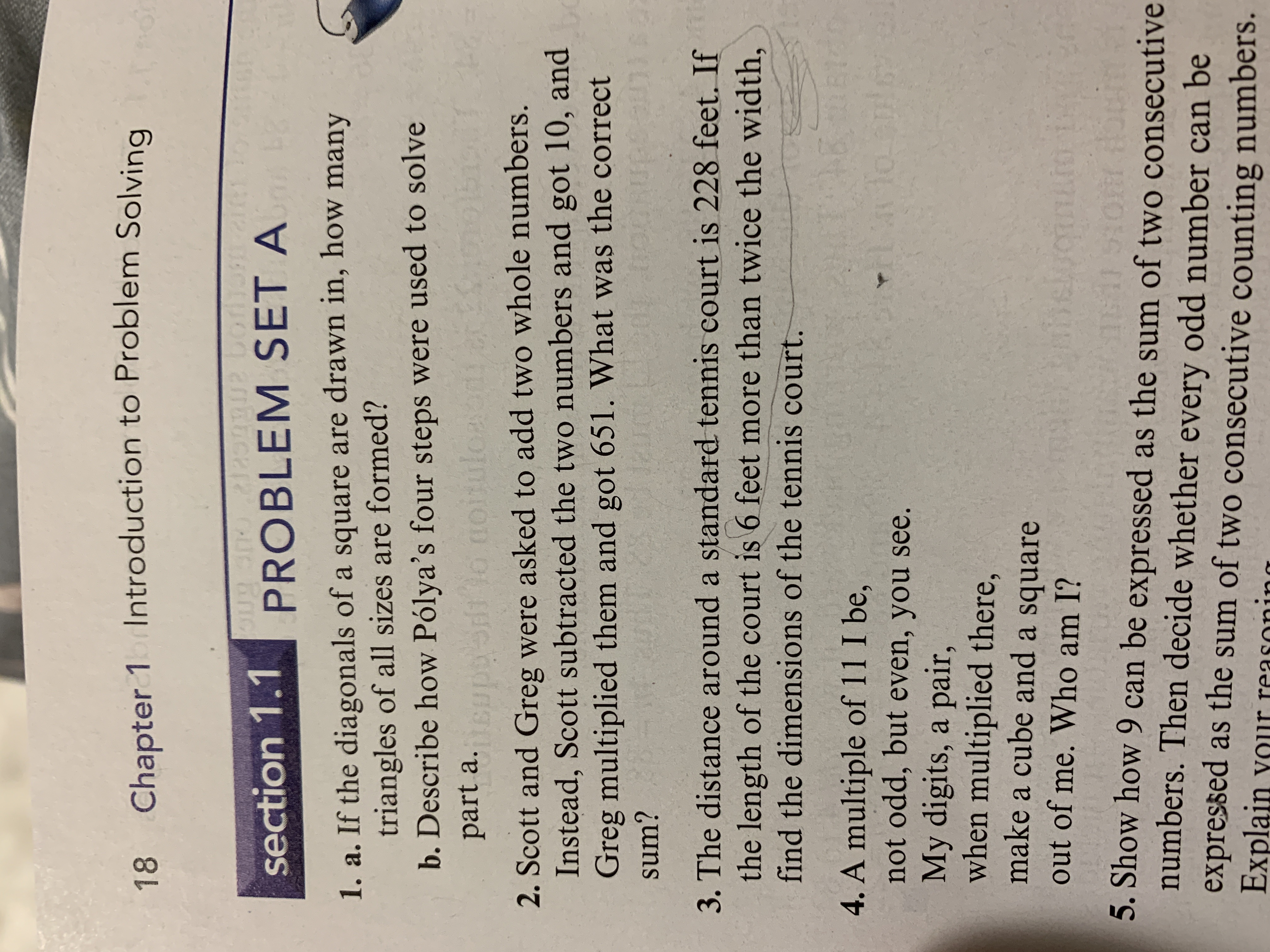# Chapter 1alntroduction to Problem Solving18Oug snsection 1.1 PROBLEM SET Al2o 9 12 bori1. a. If the diagonals of a square are drawn in, how manytriangles of all sizes are formed?b. Describe how Pólya's four steps were used to solvenoirulcaeiicppssdpart a.2. Scott and Greg were asked to add two whole numbers.Instead, Scott subtracted the two numbers and got 10, andGreg multiplied them and got 651. What was the correctJarciUTGsum?3. The distance around a standard tennis court is 228 feet. Ifthe length of the court is 6 feet more than twice the width,find the dimensions of the tennis court.C2 B14. A multiple of 11 I be,not odd, but even, you seeMy digits, a pair,when multiplied there,make a cube and a square(Ho hpleout of me. Who am I?ot tocinAOfH5. Show how 9 can be expressed as the sum of two consecutivenumbers. Then decide whether every odd number can beexpressed as the sum of two consecutive counting numbersExplain vour reasonina

Question
25 views

I need help solving number 2help_outlineImage TranscriptioncloseChapter 1alntroduction to Problem Solving 18 Oug sn section 1.1 PROBLEM SET A l2o 9 12 bori 1. a. If the diagonals of a square are drawn in, how many triangles of all sizes are formed? b. Describe how Pólya's four steps were used to solve noirulcae iicppssd part a. 2. Scott and Greg were asked to add two whole numbers. Instead, Scott subtracted the two numbers and got 10, and Greg multiplied them and got 651. What was the correct Jarci UTG sum? 3. The distance around a standard tennis court is 228 feet. If the length of the court is 6 feet more than twice the width, find the dimensions of the tennis court. C2 B1 4. A multiple of 11 I be, not odd, but even, you see My digits, a pair, when multiplied there, make a cube and a square (Ho h ple out of me. Who am I? ot tocin AOfH 5. Show how 9 can be expressed as the sum of two consecutive numbers. Then decide whether every odd number can be expressed as the sum of two consecutive counting numbers Explain vour reasonina fullscreen
check_circle

star
star
star
star
star
1 Rating
Step 1

Let x and y be the whole numbers.

Scott subtracted the two numbers and got 10. That is, xy = 10.

Greg multiplied ...

### Want to see the full answer?

See Solution

#### Want to see this answer and more?

Solutions are written by subject experts who are available 24/7. Questions are typically answered within 1 hour.*

See Solution
*Response times may vary by subject and question.
Tagged in

### Other